A sequence of numberssuch that; this class of sequences is denoted by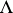and is used, in particular, in the theory of lacunary series and in the theory of lacunary trigonometric series. There are generalizations of the class. For example, the class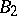: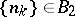if there is ansuch that the number of solutions of the equations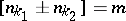(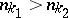and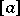is the integer part of the number) does not exceedfor any integer; the class: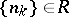if there is ansuch that the number of solutions of the equations(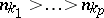) does not exceedfor any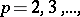and any integer; and the classes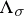,,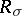, consisting of sequences that split into finitely-many sequences of the classes,,, respectively.